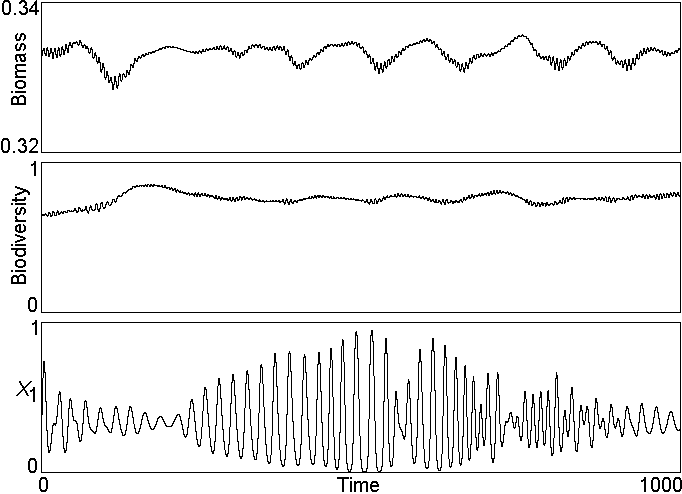# A Simple Spatiotemporal Chaotic Lotka-Volterra Model

J. C. Sprott and J. C. Wildenberg
Departments of Physics, University of Wisconsin, 1150 University Avenue, Madison, WI 53706, USA

Yousef Azizi
Institute for Advanced Studies in Basic Sciences, Zanjan, Iran

### ABSTRACT

A mathematically simple example of a high-dimensional (many-species) Lotka-Volterra model that exhibits spatiotemporal chaos in one spatial dimension is described. The model consists of a closed ring of identical agents, each competing for fixed finite resources with two of its four nearest neighbors. The model is prototypical of more complicated models in its quasiperiodic route to chaos (including attracting 3-tori), bifurcations, spontaneous symmetry breaking, and spatial pattern formation.

Ref: J. C. Sprott, J. C. Wildenberg, and Y. Azizi, Chaos Solitons & Fractals 26, 1035-1043 (2005)

The complete paper is available in PDF format.

Fig. 1. Eigenvalues of the coexisting equilibrium with N = 100 and s = 1.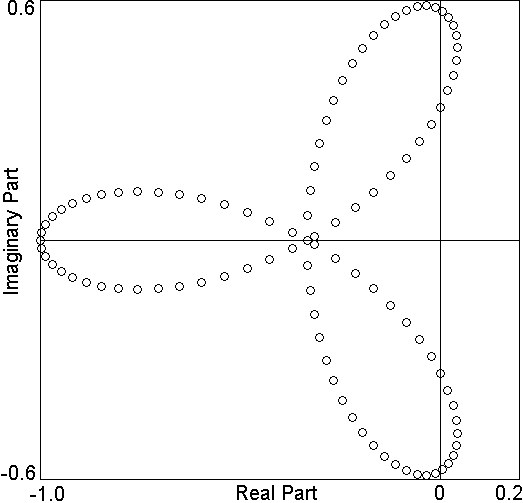Fig. 2. A one-dimensional ring of species interacting according to Eq. (2).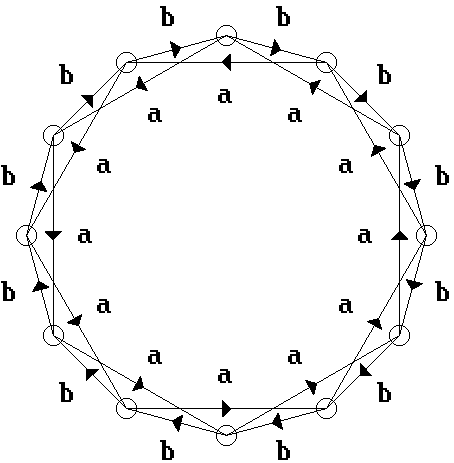Fig. 3. Largest Lyapunov exponent and Kaplan-Yorke dimension for Eq. (2) with s = 1.Fig. 4. Largest Lyapunov exponent for Eq. (2) with N = 100 showing the quasiperiodic route to chaos that is typical of high-dimensional systems.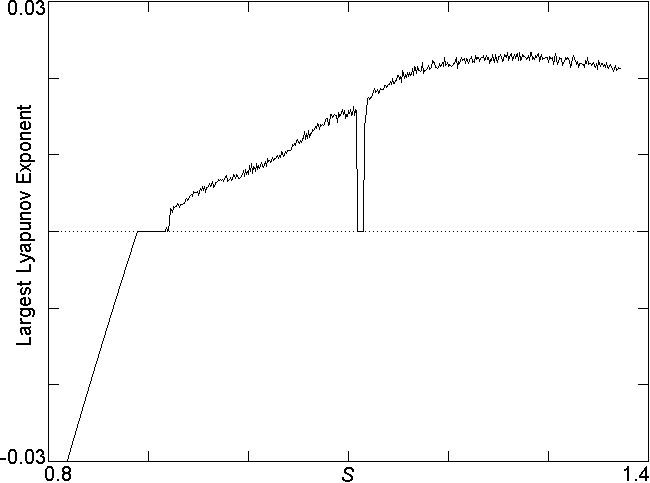Fig. 5. Spatiotemporal plot of |xi+1 - xi| from Eq. (2) with N = 480 and s = 1.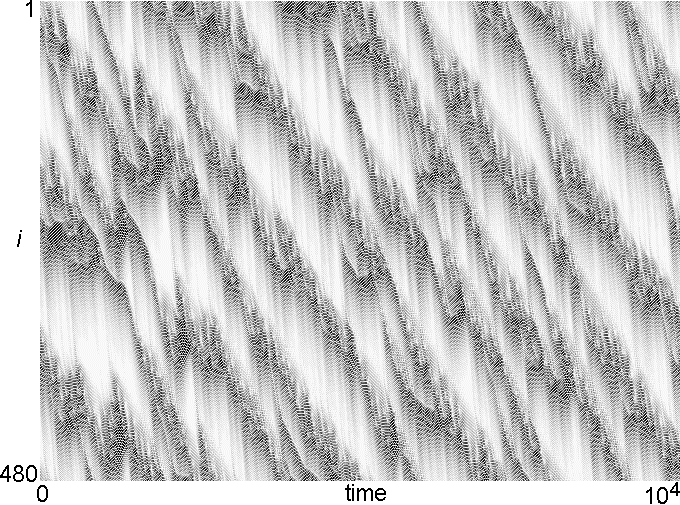Fig. 6. Spatiotemporal cross-correlation function with N = 100 and s = 1 showing propagation and dispersion.Fig. 7. Total biomass, biodiversity, and a typical x(t) versus time for Eq. (2) with N = 100 and s = 1.